Paparazzi UAS  v5.17_devel-24-g2ae834f Paparazzi is a free software Unmanned Aircraft System.
pprz_polyfit_float.h File Reference

Polynomial regression. More...This graph shows which files directly or indirectly include this file:

Go to the source code of this file.

## Functions

void pprz_polyfit_float (float *x, float *y, int n, int p, float *c)
Polynomial regression. More...

## Detailed Description

Polynomial regression.

Definition in file pprz_polyfit_float.h.

## Function Documentation

 void pprz_polyfit_float ( float * x, float * y, int n, int p, float * c )

Polynomial regression.

Polynomial regression is a form of linear regression in which the relationship between the independent variable x and the dependent variable y is modelled as an nth order polynomial.

Considering the regression model: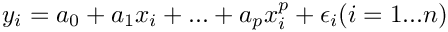in matrix form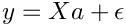where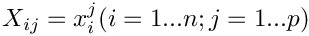The vector of estimated polynomial regression coefficients using ordinary least squares estimation is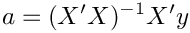Parameters
 [in] x pointer to the input array of independent variable X [n] [in] y pointer to the input array of dependent variable Y [n] [in] n number of input measurments [in] p degree of the output polynomial [out] c pointer to the output array of polynomial coefficients [p]

Polynomial regression is a form of linear regression in which the relationship between the independent variable x and the dependent variable y is modelled as an nth order polynomial.

Considering the regression model: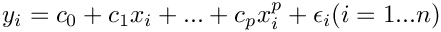in matrix form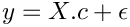whereThe vector of estimated polynomial regression coefficients using ordinary least squares estimation is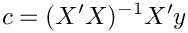Parameters
 [in] x pointer to the input array of independent variable X [n] [in] y pointer to the input array of dependent variable Y [n] [in] n number of input measurments [in] p degree of the output polynomial [out] c pointer to the output array of polynomial coefficients [p+1]

Definition at line 63 of file pprz_polyfit_float.c.Here is the call graph for this function: